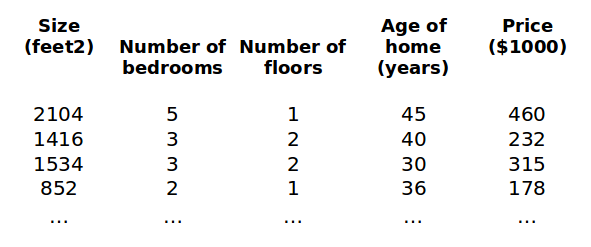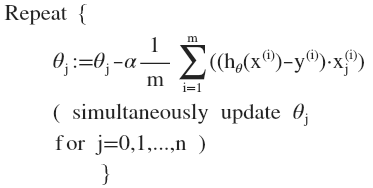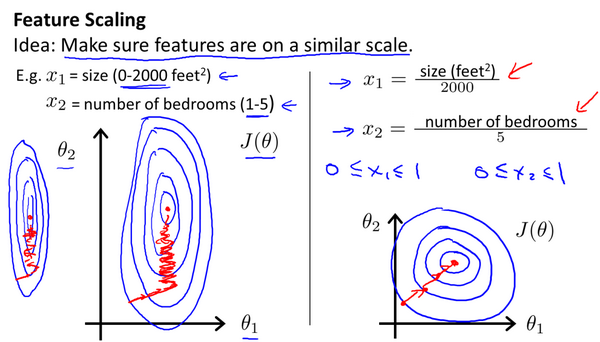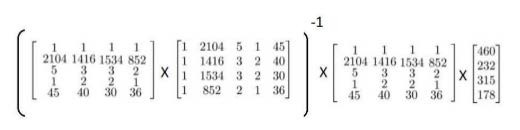># 从 0 开始机器学习 - 多维特征 & 多变量梯度下降 & 多项式回归 & 正规方程

## 一、多变量梯度下降

### 1.1 多维特征• $n$：输入特征的数量，即特征矩阵列数，也即特征向量的维度
• ${x^{\left( i \right)}}$：训练集中第 $i$ 个实例向量，就是特征矩阵的第 $i$ 行，比如列向量 ${x}^{(2)}\text{=}\begin{bmatrix} 1416\ 3\ 2\ 40 \end{bmatrix}^T$

• ${x_j}^{\left( i \right)}$：训练集中第 $i$ 个实例的第 $j$ 个特征，比如 $x_2^{\left( 2 \right)}=3$

• 使用向量方便程序编写，一句计算特征向量的代码就可以同时计算多个输入参数，因为一个特征向量中包含所有输入参数
• 使用向量方便算法执行，梯度下降算法要求参数同时更新，如果不使用向量，那更新起来非常麻烦。

### 2.1 多变量梯度下降法

• 假设函数：$h_{\theta}\left( x \right)=\theta^{T}X={\theta_{0}}+{\theta_{1}}{x_{1}}+{\theta_{2}}{x_{2}}+…+{\theta_{n}}{x_{n}}$
• 代价函数：$J \left( \theta_0, \theta_1 … \theta_n\right) = \frac{1}{2m}\sum\limits_{i=1}^m \left( h_{\theta}(x^{(i)})-y^{(i)} \right)^{2}$
• 多变量梯度下降法### 1.3 特征缩放• $x_1 = (size - 1000) / 2000$，其中 1000 是面积平均值，2000 是最大面积减最小面积。
• $x_2 = (bedrooms - 2) / 5$，其中 2 是卧室数量平均值，5 个最大卧室数量减去最小卧室数量。

### 1.4 选择学习率的小技巧## 二、多项式回归• $x_1 = (size)$：1 = 1
• $x_2 = (size)^2$：100 = 10 的平方
• $x_3 = (size)^3$：1000 = 10 的立方

## 三、正规方程法

### 3.1 基本原理$X$ 表示输入的特征矩阵，$y$ 表示每个训练实例的结果组成的向量，$\theta$ 表示待求的参数，下面来看一个实际的例子。

### 3.2 用正规方程预测房价import numpy as np

def normalEqn(X, y):
# X.T@X 等价于 X.T.dot(X)
theta = np.linalg.inv(X.T@X)@X.T@y

return theta


### 3.3 正规方程的 2 个缺陷

• 存在重复的特征变脸：用房价例子来解释就是说比如有一个特征 $x_1$ 以英尺来表示房屋尺寸，而另一个特征 $x_2$ 以米为尺寸，但是我们知道 1 米 = 3.28 英尺，所以这两个特征向量可以互相线性表示（相当于重复），则矩阵不可逆，解决方法就是找到删除重复的特征。
• 特征数量大于训练实例数量：比如有 100 个特征变量，但是只有 10 个训练数据实例，即矩阵的行数小于列数，这样的情况也会导致矩阵不可逆，不过这种情况可以使用正则化技术解决，下次介绍吧。

## 四、向量化DLonng at 03/24/20